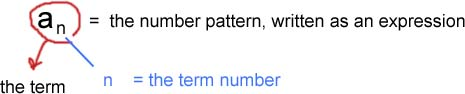Sequences

Sequences

sequence is an ordered set.  It may be finite or infinite.  Many times a sequence will follow a pattern. If  you add a constant amount to each term in order to find the next term, you are working with an arithmetic sequence.

What value is being added to each number in this sequence?

...-5, -3, -1, 1, 3, 5 ...

+2 is the constant value that is being added to each number in the sequence. It is also called the common difference, and is denoted by the variable "d."

Why is it called the common difference

If you look at the terms working backwards, you will see that the difference between term 6 and term 5 is +2; the difference between term 5 and term 4 is +2; the difference between term 3 and term 2 is +2, and so on. Thus the common difference for this sequence is +2.

A common difference is not the only relationship that numbers in a sequence can have. You'll notice other patterns. They may simply involve multiplication or division, or they may be governed by an algebraic expression, a.k.a. "rule" or "general term."

You will often see the following notation:The sequence can then be evaluated for a specific term, n, by inputting the value of n into the expression.

You may also be asked to derive the formula, or rule, from a given sequence. When you do this, you will have "produced the general term of the sequence."